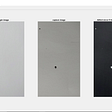# Underwater soundwave for different starting angles

Hi!

I have previously asked about a similar problem but I will try again since I have since then corrected my codes a bit and thus can not use the previous answer that I received.

The assignment is to send out and underwater sound wave at a specific depth (2000 feet) with different starting angles and let the wave travel for a distance of 25 nautical miles (25*6076 feet). I want to be able to send out different sound waves with different starting angles and for each angle plot the specific path of the sound wave.

My codes so far looks like this:

`function dZ=undervatten(x,Z)      p=[5.8796 14.4874 257.1923];      c=@(z) 4800+p(1)+(p(2)*z/1000)+(p(3)*exp(-z/1000));      c0=c(2000);      dZ=zeros(2,1);      dZ(1)=Z(2);      dZ(2)=-(c0/cosd(7.8))^2*(((-p(3)/1000)*exp(-Z(1)/1000))+p(2)/1000)/...              (4800+p(1)+p(2)*Z(1)/1000+p(3)*exp(-Z(1)/1000))^3;      end`

This is my function using the information from the assignment and I have confirmed that it is correct. The value cosd(7.8) comes from the starting angle 7.8 degrees. I am then calling this function like this:

clear all, close all, clc

`x=0:0.5:6076*25;n=7.8;[X,Z]=ode45(@undervatten,x,[2000 tand(n)]);plot(X,Z(:,1),'-r')xlabel('distance')ylabel('depth')axis ij`

This is working just fine.

Now let’s say I wanted to change n from 7.8 to -10 to 14 degrees. Hence, I want to send out waves with starting angle -10, call the function for this value, plot the curve and then move to angle -9.

Could someone please help me by showing how I can write this function so that I can call it for different values of n and getting all of the rays plotted in one graph?

Help would be much appreciated!

NOTE:-

Matlabsolutions.com provide latest MatLab Homework Help,MatLab Assignment Help for students, engineers and researchers in Multiple Branches like ECE, EEE, CSE, Mechanical, Civil with 100% output.Matlab Code for B.E, B.Tech,M.E,M.Tech, Ph.D. Scholars with 100% privacy guaranteed. Get MATLAB projects with source code for your learning and research.

`x=0:0.5:6076*25;n=-10;for i=1:25  [X,Z]=ode45(@(x,z)undervatten(x,z,n),x,[2000 tand(n)]);  Z_array(:,i)=Z(:,1);  n=n+1;endplot(x,Z_array)function dZ=undervatten(x,Z,n)p=[5.8796 14.4874 257.1923];c=@(z) 4800+p(1)+(p(2)*z/1000)+(p(3)*exp(-z/1000));c0=c(2000);dZ=zeros(2,1);dZ(1)=Z(2);dZ(2)=-(c0/cosd(n))^2*(((-p(3)/1000)*exp(-Z(1)/1000))+p(2)/1000)/...            (4800+p(1)+p(2)*Z(1)/1000+p(3)*exp(-Z(1)/1000))^3;end`

--

--

--

## More from Technical Source

Simple! That is me, a simple person. I am passionate about knowledge and reading. That’s why I have decided to write and share a bit of my life and thoughts to.

Love podcasts or audiobooks? Learn on the go with our new app.

## D4S Sunday Briefing #45## 7 Multi-label text classification dataset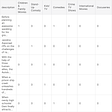## A COVID Critic’s Guide: How Much More Intense Can This Whole Thing Get?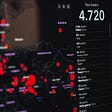## Useless datasets to explore useful concepts — part 01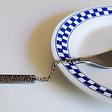## Forecasting the Future## BCH +5.50% Target price \$763.3 (next 7 days) Current price \$723.5## Make Awesome Maps in Python and Geopandas## Cereals back in fashion since COVID-19?## Technical Source

Simple! That is me, a simple person. I am passionate about knowledge and reading. That’s why I have decided to write and share a bit of my life and thoughts to.

## Could anyone help me with extracting similar features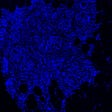## I am the beginer for doing simple image subtration to obtain defect image.